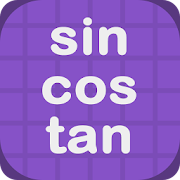# Trigonometry Table - Lumos Educational App Store3.81
Price -fREE
\$0

#### DESCRIPTION:

Search Trigonometry table by degrees, minutes, radians, sin, cos, tan, cot, sec or cosec keyword: schools, mathematics, triangles, lengths, angles, geometry, ratios, hypotenuse, perpendicular, base, cosecant (csc, cosec), secant (sec), cotangent(cot), law of sines, the law of cosines, astronomical, pure, applied, wave equation, cyclical, mechanical, electrical engineering, astronomy, navigation

#### OVERVIEW:

Trigonometry Table is a free educational mobile app By .It helps students in grades HS practice the following standards HSF.TF.A.3.

This page not only allows students and teachers download Trigonometry Table but also find engaging Sample Questions, Videos, Pins, Worksheets, Books related to the following topics.

1. HSF.TF.A.3 : Use special triangles to determine geometrically the values of sine, cosine, tangent for π/3, π/4 and π/6, and use the unit circle to express the values of sine, cosine, and tangent for x, π + x, and 2π - x in terms of their values for x, where x is any real number..

HS

#### STANDARDS:

HSF.TF.A.3

Developer:

Software Version: 1.0.2

Category:

### RELATED APPSEdSearch WebSearch